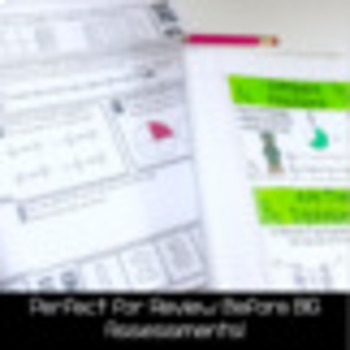# Fraction Flip Book: An Interactive Math Manipulative WITH Fraction Boot Camp3rd - 5th
Subjects
Standards
Resource Type
Formats Included
• Zip
Pages
45+

#### Also included in

1. Included in this bundle are 16 sets of task cards, activities, games, and interactive tools to help your kiddos learn about fractions. These activities range from equivalent fraction task cards to an interactive fraction flip book.The materials in this bundle are perfect for third to fourth grade, b
\$30.50
\$61.00
Save \$30.50
2. This pack includes 10-Day Boot Camp packs to help students review major math topics. There will be math Boot Camps added to this pack on various topics to help students review all concepts and skills taught. Right now there are two Boot Camps Included in this pack, each to be used over the course of
\$15.00
\$23.00
Save \$8.00

### Description

Included here is a fraction FlipBook for students and a 10-Day Fraction Flipbook. I give this to my students as a reference tool and review pack before the big test.

HERE is the Digital version of Fraction Boot Camp!

Fraction Boot Camp Includes:

This program is meant to boost students’ fraction skills, while also meeting multiple word problem and problem-solving standards. Students will participate in this program for 10 days, completing a daily review of skills.

This is perfect for a review right before your big state assessments.

*A 10 page packet for students, each including 5 questions per day.

*12 posters on ALL fraction standards.

*Pre- and post- assessments

*Directions on how to print posters to use as interactive notebook pages for students.

This will take about 15-20 minutes per day during your math block. This is meant to get students "in-shape" in terms of fraction standards!

This program is print and go, and super easy to follow. Students will learn the following skills/standards:

*Fractions on a number line

*Partitioning fractions

*Finding equivalent fractions

*Comparing fractions

*Measuring on a number line

*Fractions as part of a set

*Fractions a one whole

*Fractions as more than a whole

*Cross multiplication to find equivalent fractions

*Word Problems with fractions

The Fraction Flipbook includes the following:

After each of the fraction bar pages (as shown on the cover above), there is a page where students work on shading and understanding fraction circles, as well as fraction number lines. Fraction pages include 1/2, 1/3, 1/4, 1/6, 1/8, 1/10, 1/12.

I promise this is VERY easy to print and very easy for the kids to put together. All the kids need to do is color, cut on the dotted line of each page, and start learning!! :)

Thanks for looking and I hope your students enjoy and learn a ton!

-Dan Mault

Mr. Mault’s Marketplace

-----------------------------------------------------------------------------------------

You may also like...

Check out more Fraction Materials by Clicking Here.

Germany Flip Book

China Flip Book

Total Pages
45+
Included
Teaching Duration
2 Weeks
Report this Resource to TpT
Reported resources will be reviewed by our team. Report this resource to let us know if this resource violates TpT’s content guidelines.

### Standards

to see state-specific standards (only available in the US).
Compare two fractions with the same numerator or the same denominator by reasoning about their size. Recognize that comparisons are valid only when the two fractions refer to the same whole. Record the results of comparisons with the symbols >, =, or <, and justify the conclusions, e.g., by using a visual fraction model.
Express whole numbers as fractions, and recognize fractions that are equivalent to whole numbers. Examples: Express 3 in the form 3 = 3/1; recognize that 6/1 = 6; locate 4/4 and 1 at the same point of a number line diagram.
Recognize and generate simple equivalent fractions, (e.g., 1/2 = 2/4, 4/6 = 2/3). Explain why the fractions are equivalent, e.g., by using a visual fraction model.
Understand two fractions as equivalent (equal) if they are the same size, or the same point on a number line.
Explain equivalence of fractions in special cases, and compare fractions by reasoning about their size.